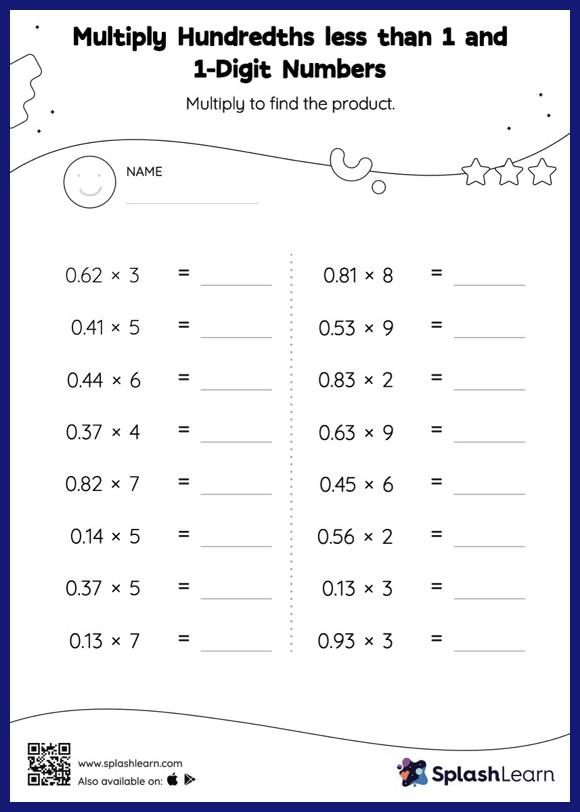# Multiply Hundredths less than 1 and 1-Digit Numbers: Horizontal Multiplication Worksheet

Home > Multiply Hundredths less than 1 and 1-Digit Numbers: Horizontal MultiplicationTask your little mathematicians to crack the code of multiplying hundredths less than 1 and 1-digit numbers with this fun worksheet. Students may omit the decimal point when multiplying a decimal by a one-digit number and then add it when writing the answer. This multiply hundredths less than 1 and 1-digit numbers worksheet gives learners many opportunities to practice this concept.